Methods of Constructing Price and Quantity Indices - Business Mathematics and Statistics

# Methods of Constructing Price and Quantity Indices - Business Mathematics and Statistics - Business Mathematics and Statistics - B Com

Index numbers may be constructed by any of the following methods—

(1) Unweighted Index :
(a) Simple Aggregative Index
(b) Simple Average of Relatives

(2) Weighted Indices:
(a) Weighted Aggregative. Index
(b) Weighted Average of Relatives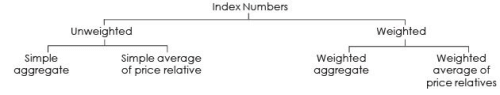UNWEIGHTED INDEX : SIMPLE AVERAGE OF PRICE RELATIVE METHOD

Under this method the price of each commodity in the current year is taken as a percentage of the price of corresponding item of the base year and the index is obtained by averaging these percentage figures.
Arithmetic mean or geometric mean may be used to average these percentages.

When arithmetic mean is used for averaging the relatives, the formula for computing the index is :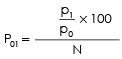Where p1 = price of current year
p0 = price of base year
N = Total Number of items

When geometric mean is used  for averaging the relatives, the formula for computing the index is :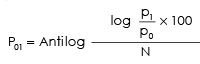Example 1 :
From the following data construct an index for 2012 taking 2011 as base by Price Relative method using
(a) Arithmetic Mean
(b) Geometric Mean for averaging relatives: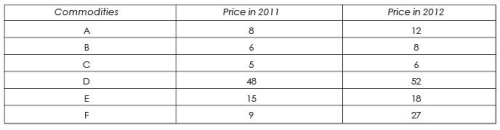Solution : Index Number using Arithmetic Mean of Price Relative

Table : Calculation of Price Relatives of Arithmetic Mean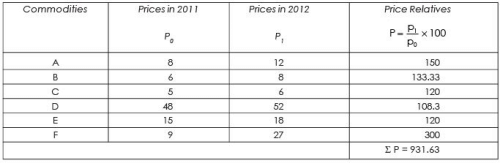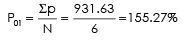To determine the value of a and b, the following two normal equations are to be solved simultaneously:

Table : Calculation of Price Relatives of Geometric Mean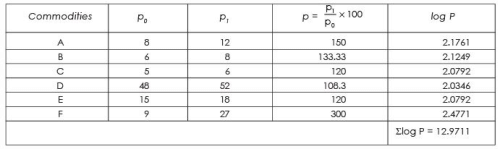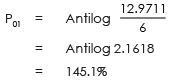Note : The difference in the answer is due to the method of averaging used.

Unweighted Indices

(a) Simple Aggregate Method - This is the simplest method of construction index numbers. It consists of expressing the aggreate price of all commodities in the current year as a per cent of the aggregate price in the base year. Symbolically: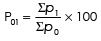Where Σp0 = total of prices of all commodities of base year
Σp1 = total of prices of all commodities of current year
p01 = Index Number of current year

Examples : 2 From the following data construct a price Index for year 2012 taking year 2009 as base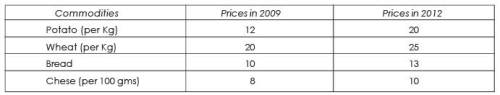Solution :
Table : Construction of Price Index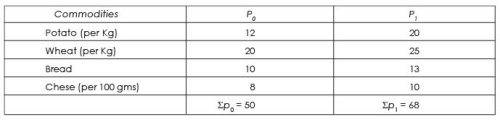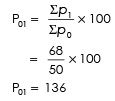This means that as compared to 2009, in 2012 there is a net increase in the prices of the given commodities to the extent of 36%.

This method has following limitations –

(1) The index is affected by the units in which the prices are quoted (such as litres, kilogram etc.). In the preceding example, if the prices of chese is taken in per kg (instead of as per 100 gms) e.g.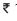80 in 2009 and100 in 2012 the index so computed would differ as follows :

Table : Construction of Price Index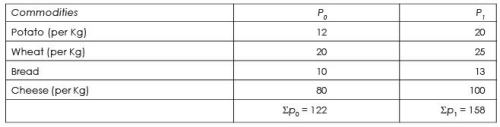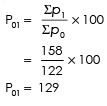The net increase in price now is only 29%.

(2) The relative importance of various commodities is not taken into account in as it is unweighted. Thus according to this method equal weights (importance) would be attached to wheat and salt in computing a cost of living index.

(3) This method is influenced by the magnitude of prices i.e. the higher the price of the commodity, the greater is the influence on the Index number. Such price quotations become the concealed weights which have no logical significance.

The document Methods of Constructing Price and Quantity Indices - Business Mathematics and Statistics | Business Mathematics and Statistics - B Com is a part of the B Com Course Business Mathematics and Statistics.
All you need of B Com at this link: B Com

115 videos|142 docs

## FAQs on Methods of Constructing Price and Quantity Indices - Business Mathematics and Statistics - Business Mathematics and Statistics - B Com

 1. What are price and quantity indices in business mathematics and statistics?Ans. Price and quantity indices in business mathematics and statistics are measures used to track the changes in prices and quantities of goods or services over time. These indices help in understanding the overall price and quantity trends in a particular market or industry.
 2. How are price indices constructed?Ans. Price indices are constructed by selecting a base year and assigning it a value of 100. The prices of various goods or services in different years are then compared to the prices in the base year. The ratio of the current year's price to the base year's price is multiplied by 100 to calculate the price index for that year.
 3. What is the purpose of constructing price indices?Ans. The purpose of constructing price indices is to measure inflation or deflation in an economy. By tracking the changes in prices over time, these indices provide valuable information about the purchasing power of consumers, the cost of living, and the overall economic health of a country.
 4. How are quantity indices calculated?Ans. Quantity indices are calculated by comparing the quantities of goods or services produced or consumed in different years to a base year. Similar to price indices, a base year is assigned a value of 100. The ratio of the current year's quantity to the base year's quantity is multiplied by 100 to calculate the quantity index for that year.
 5. What are the limitations of price and quantity indices?Ans. Price and quantity indices have certain limitations. They may not accurately capture the quality changes of goods or services over time. Additionally, the selection of the base year can affect the interpretation of the indices. Moreover, these indices do not take into account factors such as changes in consumer preferences or technological advancements, which can also impact prices and quantities.

115 videos|142 docsExplore Courses for B Com examSignup to see your scores go up within 7 days! Learn & Practice with 1000+ FREE Notes, Videos & Tests.
10M+ students study on EduRev
Track your progress, build streaks, highlight & save important lessons and more!
Related Searches

,

,

,

,

,

,

,

,

,

,

,

,

,

,

,

,

,

,

,

,

,

;